### Input Data Sets

Subsections:

You can use four different kinds of input data sets in the CALIS procedure, and you can use them simultaneously. The DATA= data set contains the data to be analyzed, and it can be an ordinary SAS data set containing raw data or a special TYPE=COV, TYPE=UCOV, TYPE=CORR, TYPE=UCORR, TYPE=SSCP, or TYPE=FACTOR data set containing previously computed statistics. The INEST= data set specifies an input data set that contains initial estimates for the parameters used in the optimization process, and it can also contain boundary and general linear constraints on the parameters. If the model does not change too much, you can use an OUTEST= data set from a previous PROC CALIS analysis; the initial estimates are taken from the values of the _TYPE_=PARMS observation. The INMODEL= or INRAM= data set contains information of the analysis models (except for user-written programming statements). Often the INMODEL= data set is created as the OUTMODEL= data set from a previous PROC CALIS analysis. See the section OUTMODEL= or OUTRAM= Data Set for the structure of both OUTMODEL= and INMODEL= data sets. Using the INWGT= data set enables you to read in the weight matrix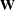that can be used in generalized least squares, weighted least squares, or diagonally weighted least squares estimation.

#### BASEFIT= or INBASEFIT= Data Set

The BASEFIT= or INBASEFIT= data set saves the fit function value and the degrees of freedom of a baseline model for computing various fit indices, especially the incremental fit indices. Typically, the BASEFIT= data set is created as an OUTFIT= data set from a previous PROC CALIS fitting of a customized baseline model. See the section OUTFIT= Data Set for details about the format of the OUTFIT= and BASEFIT= data sets.

#### DATA= Data Set

A TYPE=COV, TYPE=UCOV, TYPE=CORR, or TYPE=UCORR data set can be created by the CORR procedure or various other procedures. It contains means, standard deviations, the sample size, the covariance or correlation matrix, and possibly other statistics depending on which procedure is used.

If your data set has many observations and you plan to run PROC CALIS several times, you can save computer time by first creating a TYPE=COV, TYPE=UCOV, TYPE=CORR, or TYPE=UCORR data set and using it as input to PROC CALIS.

For example, assuming that PROC CALIS is first run with an OUTMODEL=MODEL option, you can run the following statements in subsequent analyses with the same model in the first run:

/* create TYPE=COV data set */
proc corr cov nocorr data=raw outp=cov(type=cov);
run;
/* analysis using correlations */
proc calis corr data=cov inmodel=model;
run;
/* analysis using covariances */
proc calis data=cov inmodel=model;
run;


Most procedures automatically set the TYPE= option of an output data set appropriately. However, the CORR procedure sets TYPE=CORR unless an explicit TYPE= option is used. Thus, (TYPE=COV) is needed in the preceding PROC CORR request, since the output data set is a covariance matrix. If you use a DATA step with a SET statement to modify this data set, you must declare the TYPE=COV, TYPE=UCOV, TYPE=CORR, or TYPE=UCORR attribute in the new data set.

You can use a VAR statement with PROC CALIS when reading a TYPE=COV, TYPE=UCOV, TYPE=CORR, TYPE=UCORR, or TYPE=SSCP data set to select a subset of the variables or change the order of the variables.

Caution: Problems can arise from using the CORR procedure when there are missing data. By default, PROC CORR computes each covariance or correlation from all observations that have values present for the pair of variables involved (pairwise deletion). The resulting covariance or correlation matrix can have negative eigenvalues. A correlation or covariance matrix with negative eigenvalues is recognized as a singular matrix in PROC CALIS, and you cannot compute (default) generalized least squares or maximum likelihood estimates. You can specify the RIDGE option to ridge the diagonal of such a matrix to obtain a positive definite data matrix. If the NOMISS option is used with the CORR procedure, observations with any missing values are completely omitted from the calculations (listwise deletion), and there is no possibility of negative eigenvalues (but there is still a chance for a singular matrix).

PROC CALIS can also create a TYPE=COV, TYPE=UCOV, TYPE=CORR, or TYPE=UCORR data set that includes all the information needed for repeated analyses.

If the data set DATA=RAW does not contain missing values, the following statements should give the same PROC CALIS results as the previous example:

/* using correlations */
proc calis corr data=raw outstat=cov inmodel=model;
run;
/* using covariances */
proc calis data=cov inmodel=model;
run;


You can create a TYPE=COV, TYPE=UCOV, TYPE=CORR, TYPE=UCORR, or TYPE=SSCP data set in a DATA step. Be sure to specify the TYPE= option in parentheses after the data set name in the DATA statement and include the _TYPE_ and _NAME_ variables. If you want to analyze the covariance matrix but your DATA= data set is a TYPE=CORR or TYPE=UCORR data set, you should include an observation with _TYPE_=STD giving the standard deviation of each variable. By default, PROC CALIS analyzes the recomputed covariance matrix even when a TYPE=CORR data set is provided, as shown in the following statements:

data correl(type=corr);
input _type_ $_name_$ X1-X3;
datalines;
std   .   4.  2.  8.
corr  X1  1.0  .   .
corr  X2   .7 1.0  .
corr  X3   .5  .4 1.0
;
proc calis inmodel=model;
run;


#### INEST= Data Set

You can use the INEST= (or INVAR=) input data set to specify the initial values of the parameters used in the optimization and to specify boundary constraints and the more general linear constraints that can be imposed on these parameters.

The variables of the INEST= data set must correspond to the following:

• a character variable _TYPE_ that indicates the type of the observation

• n numeric variables with the parameter names used in the specified PROC CALIS model

• the BY variables that are used in a DATA= input data set

• a numeric variable _RHS_ (right-hand side); needed only if linear constraints are used

• additional variables with names corresponding to constants used in the programming statements

The content of the _TYPE_ variable defines the meaning of the observation of the INEST= data set. PROC CALIS recognizes observations with the following _TYPE_ specifications.

PARMS

specifies initial values for parameters that are defined in the model statements of PROC CALIS. The _RHS_ variable is not used. Additional variables can contain the values of constants that are referred to in programming statements. At the beginning of each run of PROC CALIS, the values of the constants are read from the PARMS observation for initializing the constants in the SAS programming statements.

UPPERBD | UB

specifies upper bounds with nonmissing values. The use of a missing value indicates that no upper bound is specified for the parameter. The _RHS_ variable is not used.

LOWERBD | LB

specifies lower bounds with nonmissing values. The use of a missing value indicates that no lower bound is specified for the parameter. The _RHS_ variable is not used.

LE | <= | <

specifies the linear constraint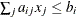. The n parameter values contain the coefficients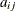, and the _RHS_ variable contains the right-hand-side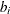. The use of a missing value indicates a zero coefficient.

GE | >= | >

specifies the linear constraint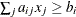. The n parameter values contain the coefficients, and the _RHS_ variable contains the right-hand-side. The use of a missing value indicates a zero coefficient.

EQ | =

specifies the linear constraint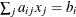. The n parameter values contain the coefficients, and the _RHS_ variable contains the right-hand-side. The use of a missing value indicates a zero coefficient.

The constraints specified in the INEST=, INVAR=, or ESTDATA= data set are added to the constraints specified in BOUNDS and LINCON statements.

You can use an OUTEST= data set from a PROC CALIS run as an INEST= data set in a new run. However, be aware that the OUTEST= data set also contains the boundary and general linear constraints specified in the previous run of PROC CALIS. When you are using this OUTEST= data set without changes as an INEST= data set, PROC CALIS adds the constraints from the data set to the constraints specified by a BOUNDS and LINCON statement. Although PROC CALIS automatically eliminates multiple identical constraints, you should avoid specifying the same constraint a second time.

#### INMODEL= or INRAM= Data Set

This data set is usually created in a previous run of PROC CALIS. It is useful if you want to reanalyze a problem in a different way such as using a different estimation method. You can alter an existing OUTMODEL= data set in the DATA step to create the INMODEL= data set that describes a modified model. See the section OUTMODEL= or OUTRAM= Data Set for more details about the INMODEL= data set.

#### INWGT= Data Set

This data set enables you to specify a weight matrix other than the default matrix for the generalized, weighted, and diagonally weighted least squares estimation methods. If you also specify the INWGTINV option (or use the INWGT(INV)=option), the INWGT= data set is assumed to contain the inverse of the weight matrix, rather than the weight matrix itself. The specification of any INWGT= data set for unweighted least squares or maximum likelihood estimation is ignored. For generalized and diagonally weighted least squares estimation, the INWGT= data set must contain a _TYPE_ and a _NAME_ variable as well as the manifest variables used in the analysis. The value of the _NAME_ variable indicates the row index i of the weight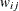. For weighted least squares, the INWGT= data set must contain _TYPE_, _NAME_, _NAM2_, and _NAM3_ variables as well as the manifest variables used in the analysis. The values of the _NAME_, _NAM2_, and _NAM3_ variables indicate the three indices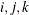of the weight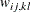. You can store information other than the weight matrix in the INWGT= data set, but only observations with _TYPE_=WEIGHT are used to specify the weight matrix. This property enables you to store more than one weight matrix in the INWGT= data set. You can then run PROC CALIS with each of the weight matrices by changing only the _TYPE_ observation in the INWGT= data set with an intermediate DATA step.

See the section OUTWGT= Data Set for more details about the INWGT= data set.